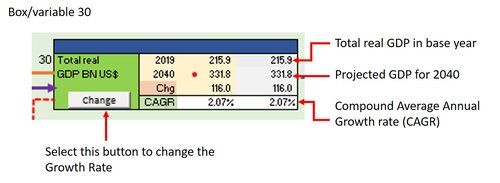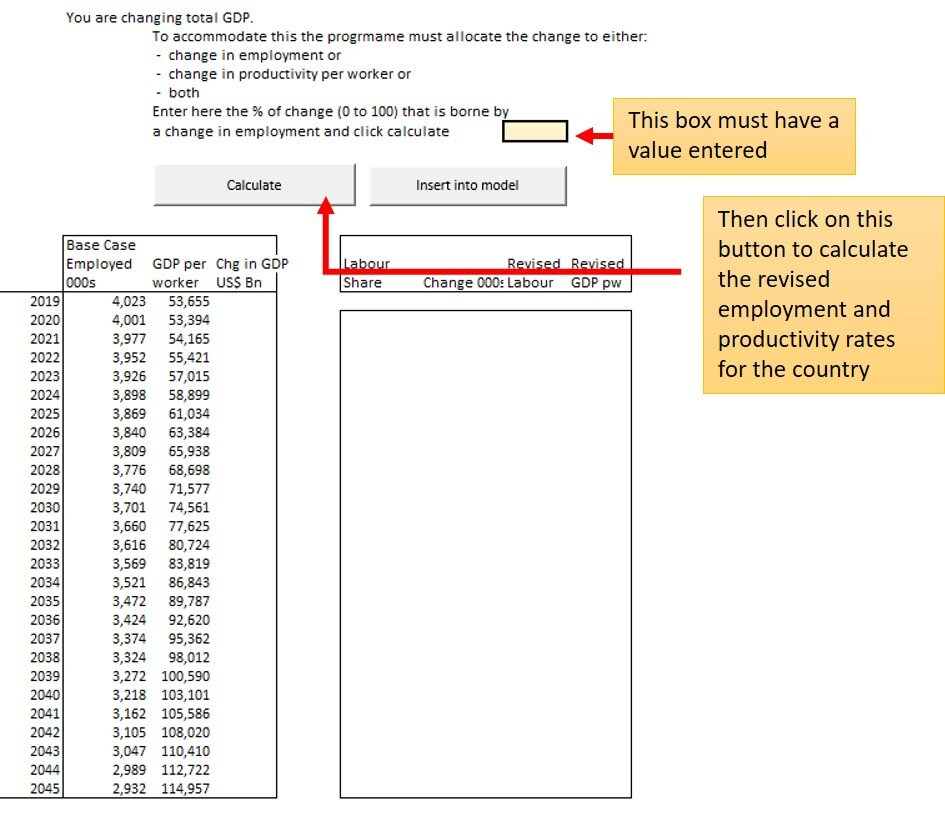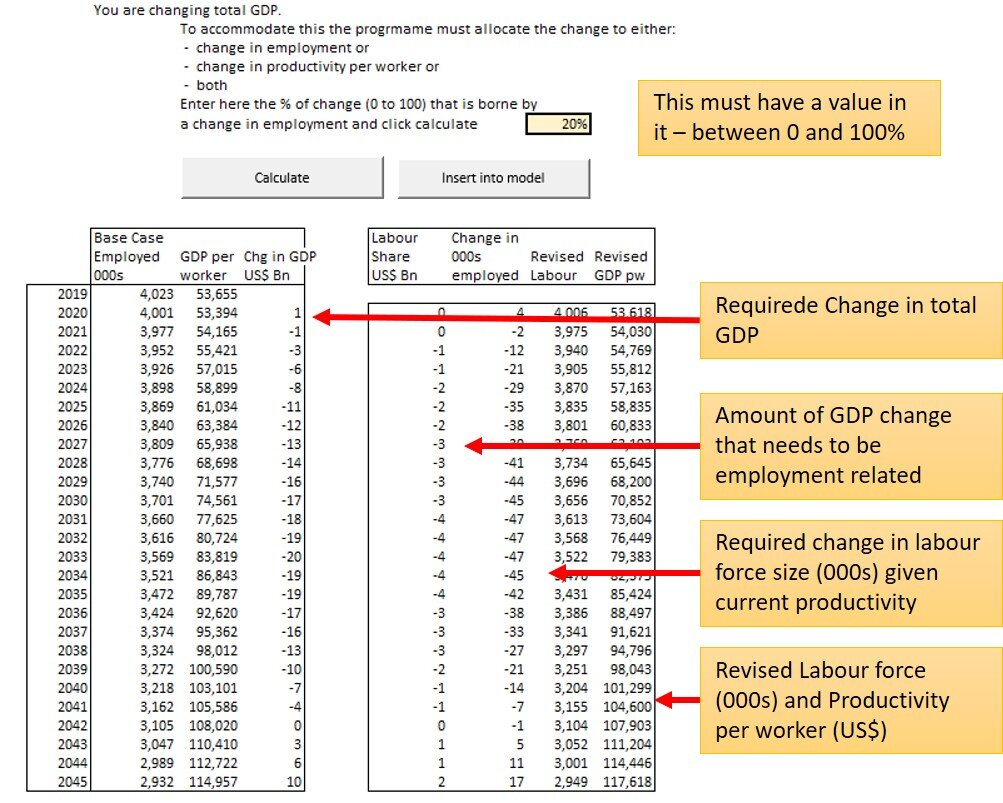### Step Two (B) Changing a GDP ForecastThe previous section detailed how to change individual determinant variables. However, there are instances where expected change in a dependent variable is known - but the components of it.

Such an instance is total Real GDP growth.

There will be instances where the question is “what if the GDP grows at a faster/slower rate?”

To answer the question by using the determinant variables the user would need to alter a combination of

• propensity to be employed by age and gender
• Fixed Capital Investment
• Productivity trend
• and if really long term - education index

experimenting with different values to get the right GDP growth rate.

To make this process easier we have added the ability to change GDP growth rate and the relative impact of it on employment and productivity and the program works out the required change in the explanatory variables to produce that growth rate..

This means the user can quickly see the impact of a change in GDP growth on:

• employment
• wages
• Household incomes
• Household expenditure

#### TO CHANGE GDP GROWTH

Select the Box for Total Real GDP (as shown on the right here).

Note that the values in the two data columns are US\$ Bns and the CAGR is for the years 2019 to 2040.Click on the ‘Change’ button and you are taken to a new window (see below) showing the value of that variable under the base case from 2005 to 2045. The data for years 2005 to 2019 are actual as reported by that country. Values for subsequent years are the current forecast under the Global Demographics Ltd.’s master model.

However, in this instance there is an additional column shown - YOY% (year on year percentage growth rate). Also note the additional tables on the right (not shown here, but shown below) - they are explained below.

As for other determinant variables you click on the clear button and this will clear the ‘revised’ and ‘% YOY’ columns and then enter for the years you wish the % YOY growth. You need not enter all years as the button below that fills in the gaps in a linear fashion.

Clicking the ‘Fill in new data series’ button then completes the % YOY column and also calculates the revised column values.After clicking on the ‘Fill in New data series’ button shift to the tables on the right as shown below.

The two data columns show the current values for total employed and GDP per worker - which must change if the GDP is to be changed. (total GDP equals total employed x output per worker).

The yellow box is where the user enters a value for the proportion of the change that is achieved by altering the level fo employment (with the balance by changes in productivity). For example if employment grows faster than GDP growth then productivity per worker must decrease.

In times of crisis/change countries react very differently on this. In the Global Financial Crash (2008) Germany experienced no decline in total employed, but did experience a decline in output per worker (many were on subsidised salaries during the period). In contrast the USA suffered relatively small decline in productivity per worker but a significant decline in the number of persons employed. In fact for the USA 77% of the reduction in total GDP was a function of a reduction in employment.Enter a value in the yellow box (in this example we have entered 20%) and press the calculate button.

This results in the tables being completed. Specifically

• Absolute change in GDP is shown (column 3)
• The amount of that which must be attributed to a change in number of employed (Column 4)
• The change in number of employed given current productivity (000s persons) (Column 5)
• Revised total Labour force and revised GDP per worker (which compare to columns 1 and 2 respectively)

You can change the value entered in the yellow box and press calculate as often as needed.Finally, press the button labelled ‘insert into model’ and the program will update the relevant variables (see below) and return you to the main model screen.

Note the program alters the following variables:

Labour force Participation rates (Boxes 12 to 14) so that the total employed equals the target figure for the year.

Productivity (Box 28) so that GDP per worker is adjusted to the required figure for each year. It is necessary to use this override ‘Productivity’ figure as the key medium term drivers of productivity are accumulated Fixed Capital Investment per worker and education- neither of which change in the short-term in reality. So it is inappropriate to alter them here as well. This applies whether the GDP is being accelerated (better trade terms??) or reduced (Global Pandemic). Such events change over night the value of what the worker produces and that has to be handled using an intervening variable such as this.

The changes it makes to those two variable will result in total GDP matching the target rate of the user.

These changes also alter a number of other variables which will be highlighted when the scenario is run. Specifically

• Total employed
• Employed per household
• Dependency ratio
• Wages as a percent of GDP
• Wage per worker
• Household income (total and distribution)
• Household expenditure (total and distribution)

### By 2045

The total population of China is projected to decline to 1.378 billion persons - down from 1.411 in 2020 (Census). This assumes the average birth rate per thousand women aged 15 to 49 increases from 44 (in 2019) to 50 in 2024 and then declines to 46 by 2045, reflecting trends in improved education and affluence.

Annual births in 2024 are expected to be 15.7 million and are projected to decline to 12.4 million by 2035 and 11.541 million by 2045.

For more information on births in China, see our Special report on this topic